﻿ 基于改进粒子滤波的AUV海底地形辅助定位方法
«上一篇文章快速检索 高级检索

 智能系统学报2020, Vol. 15Issue (3): 553-559  DOI: 10.11992/tis.2019030270

### 引用本文HAN Yue, CHEN Pengyun, SHEN Peng. Seabed terrain-aided positioning method based on improved particle filtering for AUVs[J]. CAAI Transactions on Intelligent Systems, 2020, 15(3): 553-559. DOI: 10.11992/tis.201903027.### 文章历史

1. 太原旅游职业学院 现代教育信息中心，山西 太原 030032;
2. 中北大学 机电工程学院，山西 太原 030051;
3. 国家深海基地管理中心，山东 青岛 266237

Seabed terrain-aided positioning method based on improved particle filtering for AUVs
HAN Yue 1,2, CHEN Pengyun 2, SHEN Peng 3
1. Modern Education Information Centre, Taiyuan Tourism College, Taiyuan 030032, China;
2. College of Mechatronic Engineering, North University of China, Taiyuan 030051, China;
3. National Deep Sea Centre, Qingdao 266237, China
Abstract: Focusing on the seabed terrain-aided navigation of autonomous underwater vehicle (AUV), a terrain-aided positioning method based on the particle filtering method is proposed in this study. To solve the particle depletion problem of the particle filtering method, the auxiliary sampling technology is introduced. Then, a terrain-aided positioning method based on the auxiliary sampling particle filtering method, which can reduce the loss of particle diversity caused by resampling, is proposed. Simulation tests based on the semi-physical test platform show that the proposed method has high terrain positioning accuracy and strong adaptability to terrain features, which can meet the demand of AUV navigation.
Key words: autonomous underwater vehicle    underwater environment    multi-beam sounding    terrain-aided positioning    Bayesian estimation    particle filter    auxiliary sampling    semi-physical simulation

1 地形辅助定位算法模型 1.1 地形辅助定位的Bayesian估计

 ${{{X}}_{t + 1}} = {{{X}}_t} + {{{U}}_t} + {\nu _t}$
 ${{{Y}}_t} = {{{H}}_t}\left( {{{{x}}_t}} \right) + {{{E}}_t}$ (1)

 ${{{y}}_t} = {{{h}}_t}({{{x}}_t}) + {{{e}}_t}$

 $p\left( {{y}_{t}}|{{x}_{t}} \right)=\frac{1}{{{\left( 2{\text{π}} {{\sigma }^{2}} \right)}^{\frac{N}{2}\;}}}\exp \left[ -\frac{1}{2{{\sigma }^{2}}}\sum\limits_{k=1}^{N}{{{\left( {{y}_{k}}\left( {{x}_{0}} \right)-{{h}_{k}}\left( {{x}_{t}} \right) \right)}^{2}}} \right]$ (2)

 $p\left( {{x_t}|{y_t}} \right) = \frac{{p\left( {{y_t}|{x_t}} \right)p\left( {{x_t}} \right)}}{\int_{{x_t} \in {S_t}} {} p\left( {{y_t}|{x_t}} \right)p\left( {{x_t}} \right){\rm{d}}{x_t}}$

 $\begin{array}{c} p\left( {{x_t}|{Y_t}} \right) = {\alpha _t} p\left( {{x_t}|{Y_{t - 1}}} \right)p\left( {{y_t}|{x_t}} \right)= \\ \;\;\;\;\;\;\;\;\;\dfrac{{p\left( {{x_t}|{Y_{t - 1}}} \right)p\left( {{y_t}|{x_t}} \right)}}{{\displaystyle\int_{{x_t} {_t \in {S_t}}} {} {p\left( {{x_t}|{Y_{t - 1}}} \right){p_{{\nu _t}}}\left( {{x_{t + 1}} - {x_t} - {u_t}} \right)} {\text{d}} {x_t}}} \end{array}$ (3)

 $\begin{array}{c} p({X_t}|{Y_{t - 1}}) = p({x_t}|{X_{t - 1}},{Y_{t - 1}})=\\ \;\; \;\displaystyle\int_{{x_t} \in {S_{t - 1}}} {p({X_{t - 1}}|{Y_{t - 1}})p({x_t}|{x_{t - 1}}){\rm{d}}{x_{t - 1}}} = \\ \;\; \displaystyle\int_{{x_t} \in {S_{t - 1}}} {p({X_{t - 1}}|{Y_{t - 1}})({x_t} - {x_{t - 1}} - {u_{t - 1}}){\rm{d}}{x_{t - 1}}} \end{array}$ (4)

Bayesian滤波的最大困难是PDF的数值解法，即使对于线性高斯系统，其求解仍然面临着高维积分问题，计算难度较大。在Bayesian滤波的数值解法中，PF算法作为一种Bayesian滤波的近似最优方法得到了越来越多的关注，在解决这类问题上有着良好的实用性。基于多波束测探的AUV海底地形辅助导航示意如图1所示。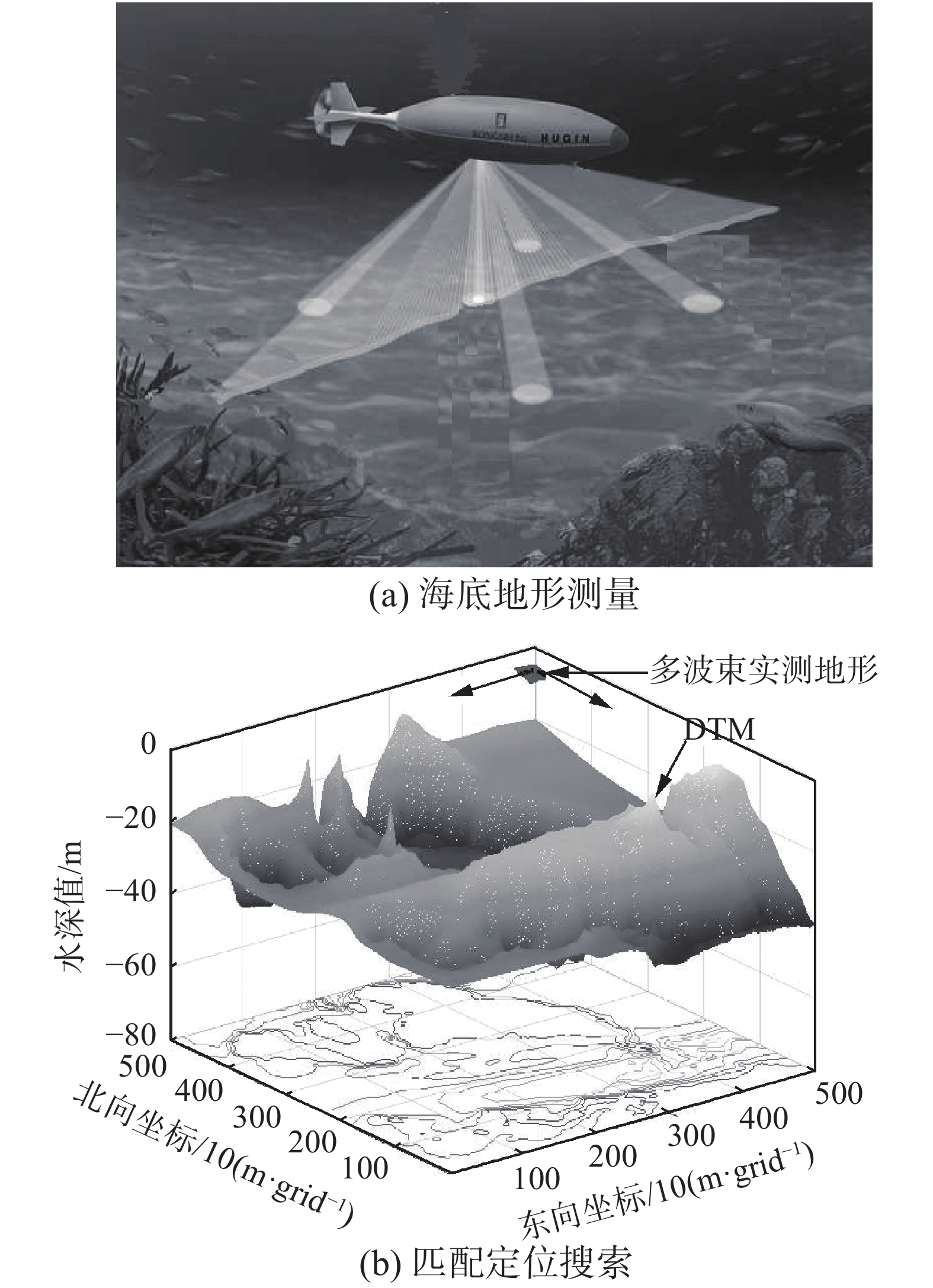Download: 图 1 基于多波束测深的AUV海底地形辅助导航示意 Fig. 1 Schematic diagram of terrain-aided navigation using multi-beam sounding
1.2 PF地形辅助定位算法

PF算法的本质是将连续积分运算变为随机样本集的求和运算，即系统状态的后验PDF可用一组在状态空间内随机抽取的加权粒子表示为

 $p({X_t}|{Y_t}) \approx \frac{1}{{{N_s}}}\sum\limits_{i = 1}^{{N_s}} {\delta ({X_t} - X_t^i)}$

 $p({X_t}|{Y_t}) \approx \sum\limits_{i = 1}^{{N_s}} {w_t^i\delta ({X_t} - X_t^i)}$

 $w_t^i = w_{t - 1}^i\frac{{p\left( {{z_t}|x_{t - 1}^i} \right)p\left( {x_t^i|x_{t - 1}^i} \right)}}{{q\left( {x_t^i|x_{t - 1}^i,{y_t}} \right)}}$ (5)

 ${\tilde {{x}}_t} = \frac{1}{{{N_j}}}\sum\limits_{i = 1}^{N_c^j} {w_t^i} x_t^i$

 ${p_t} = \sum\limits_{j = 1}^{{N_j}} {\sum\limits_{i = 1}^{N_c^j} {w_t^i(} {{x}}_t^i - {{\tilde {{x}}}_t}){{({{x}}_t^i - {{\tilde {{x}}}_t})}^{\text{T}}}}$

1.3 辅助重采样技术

1) 计算粒子滤波中的有效粒子个数 ${N_{{\text{eff}}}}$ ${N_{{\text{eff}}}}$ 的计算公式为

 ${N_{{\text{eff}}}} = {1 / {\sum\limits_{i = 1}^{{N_s}} {{{\left( {w_t^i} \right)}^2}} }}$ (6)

2) 若 ${N_{{\text{eff}}}}$ 小于设定的重采样阈值 ${N_{{\text{th}}}}$ ，从粒子集中根据重要性权值重新采样得到新的粒子集 $\left\{ {{{{x}}^*}_t^i} \right\},i = 1,2, \cdots ,N$ ，记录其父代粒子及其权值的集合 $\left\{ {{{x}}_t^j,{{w}}_t^j} \right\}_{j = 1}^M,j = 1,2, \cdots ,M$ ，以及每个父代粒子复制的次数 $\left\{ {{{N}}_c^j} \right\}_{j = 1}^M,j = 1,2, \cdots ,M$ 。其中M为父代粒子的个数。

3) 根据每个父代粒子的分布 $p(x_i^j)$ ，重新采样 $N_c^j$ 次，计算采样后粒子的权值：

 $w_t^j = \dfrac{{p({y_t}|x_{t - 1}^i)}}{{p({y_t}|x_t^*)}}$

2 地形辅助定位算法流程

1) 初始化： $t = 0$ ，根据 $p\left( {{x_0}} \right)$ 的分布采样得到粒子数为N的粒子集 $\left\{ {{{x}}_0^i} \right\}_{i = 1}^N,i = 1,2, \cdots ,N$ ，粒子的初始重要性权值为 $w_0^i = {1 / N}$

2) 重要性权值计算： $t = t + 1$ ，根据先验PDF采样得到 $x_t^i \sim q\left( {{x_t}|x_{t - 1}^i,{y_{t - 1}}} \right)$ $i = 1,2, \cdots ,N$ ，根据式(5)计算重要性权值 $w_t^i$

3) 重要性权值归一化： ${w^*}_t^i = {{w_t^i} / {\displaystyle\sum\limits_{j = 1}^N {w_t^j} }}$

4) 根据式(6)计算有效粒子个数 ${N_{{\text{eff}}}}$

5) 若 ${N_{{\text{eff}}}}$ 小于重采样阈值 ${N_{{\text{th}}}}$ ，执行辅助重采样计算；

6) 输出地形匹配的状态估计和方差估计；

7) 判断滤波是否收敛，若滤波收敛，输出定位结果；若滤波未收敛转到步骤2)。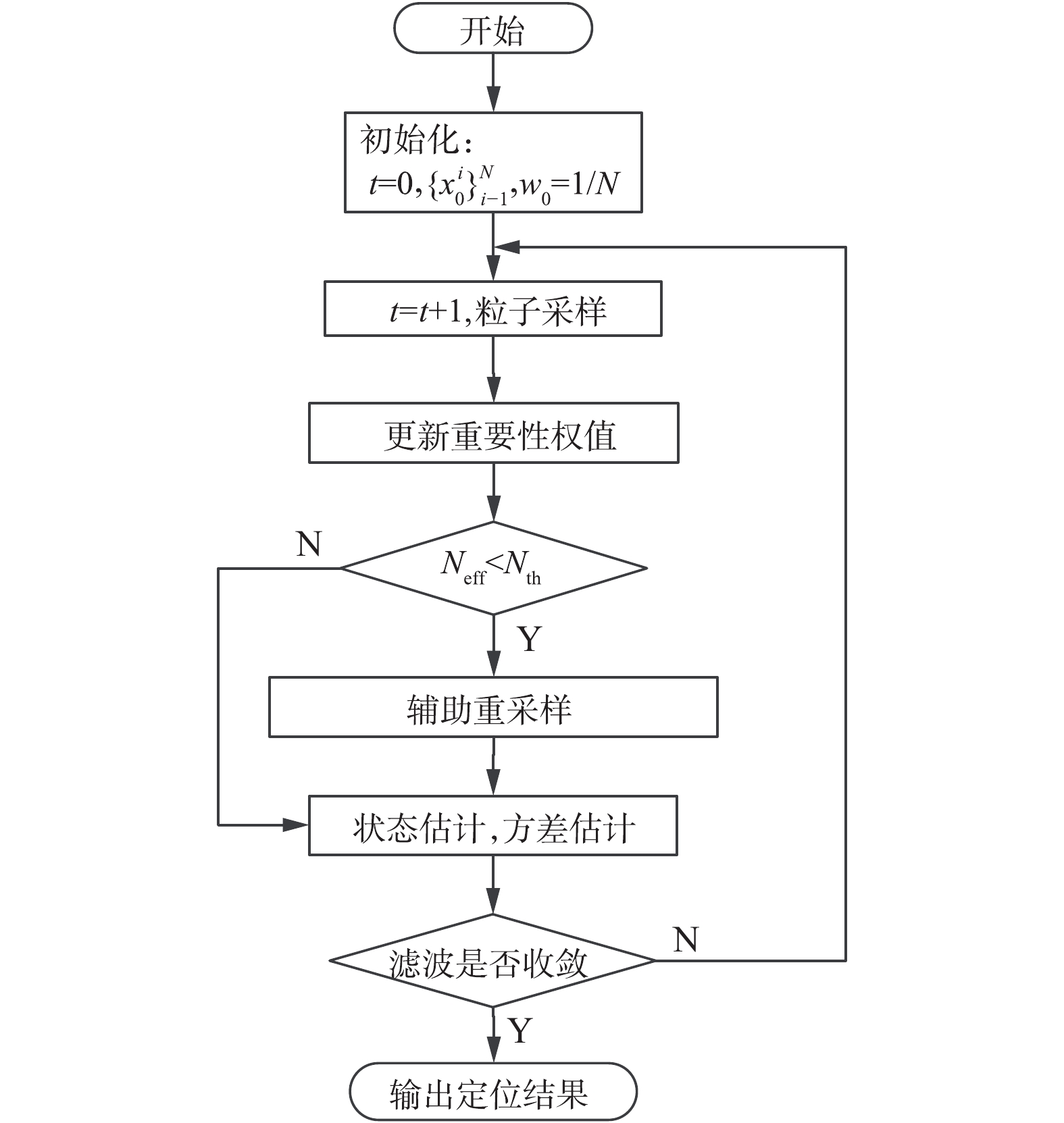Download: 图 2 算法流程 Fig. 2 Algorithm flowchart
3 仿真实验与结果分析 3.1 半物理测试平台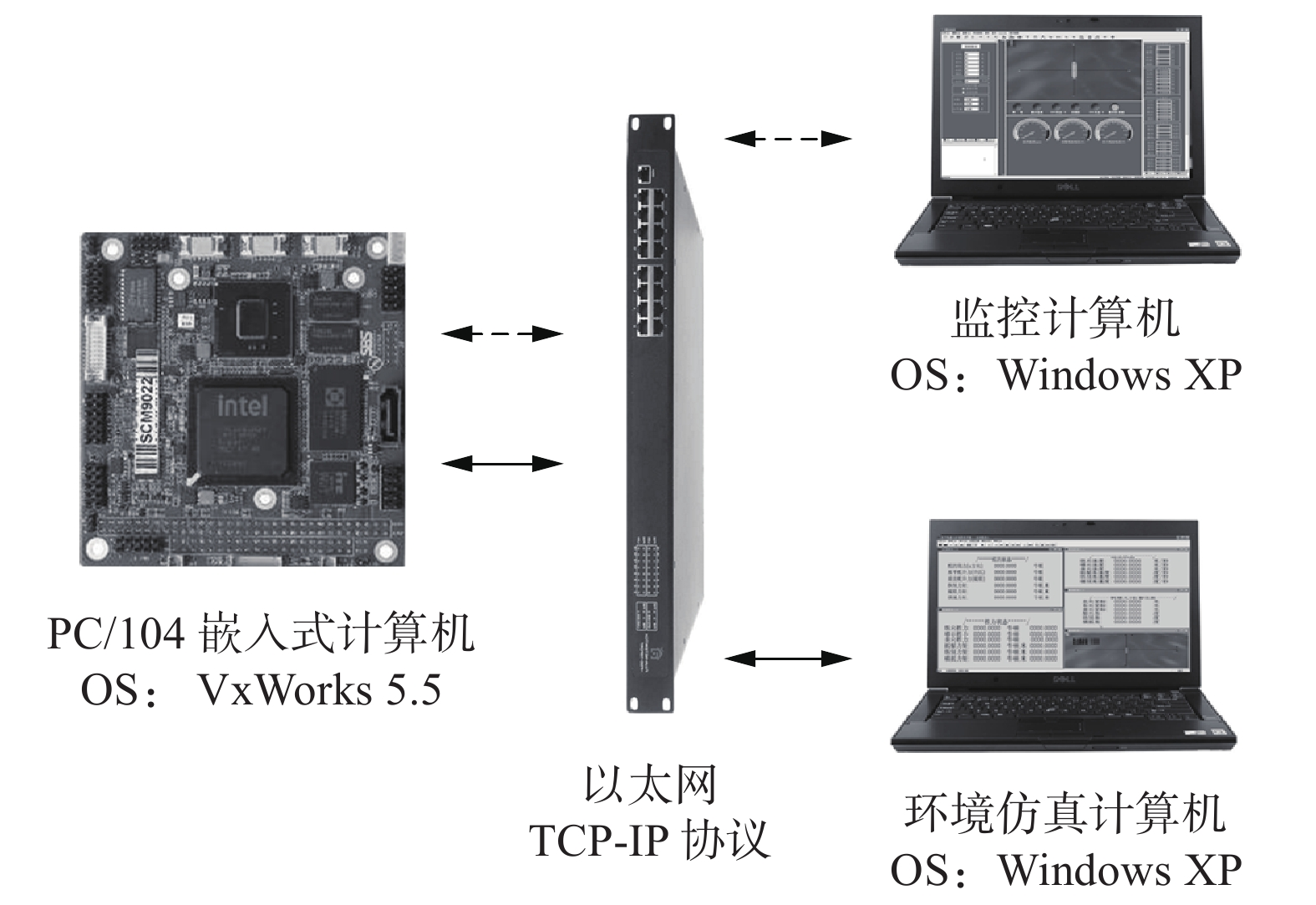Download: 图 3 半物理测试平台体系结构 Fig. 3 Structure of semi-physical test platform

1) 基于电子海图数据的算法验证；

2) 使用真实多波束测深数据的回放式仿真。

3.2 算法验证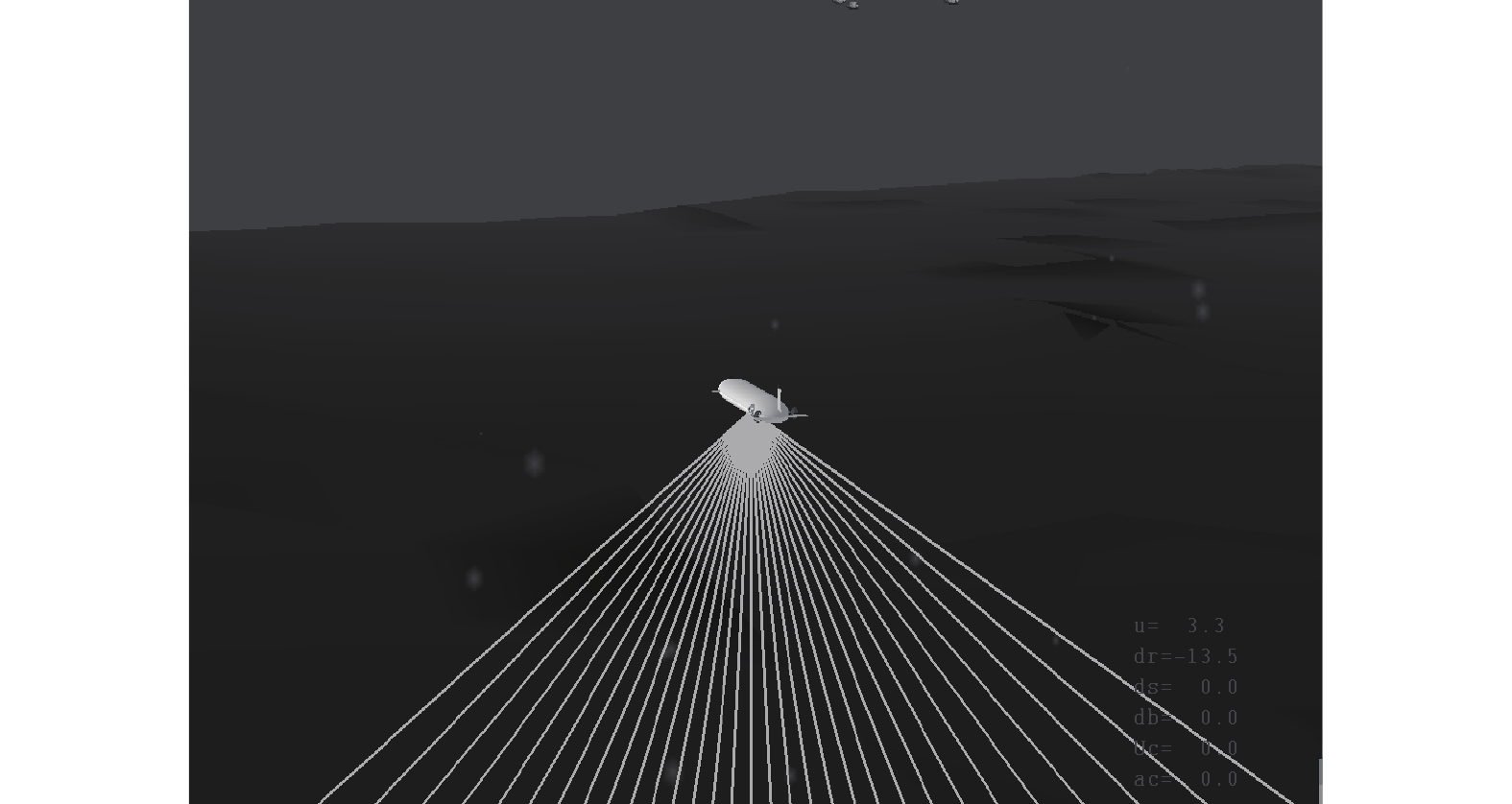Download: 图 4 Vega视景仿真界面 Fig. 4 Visual simulation interface of Vega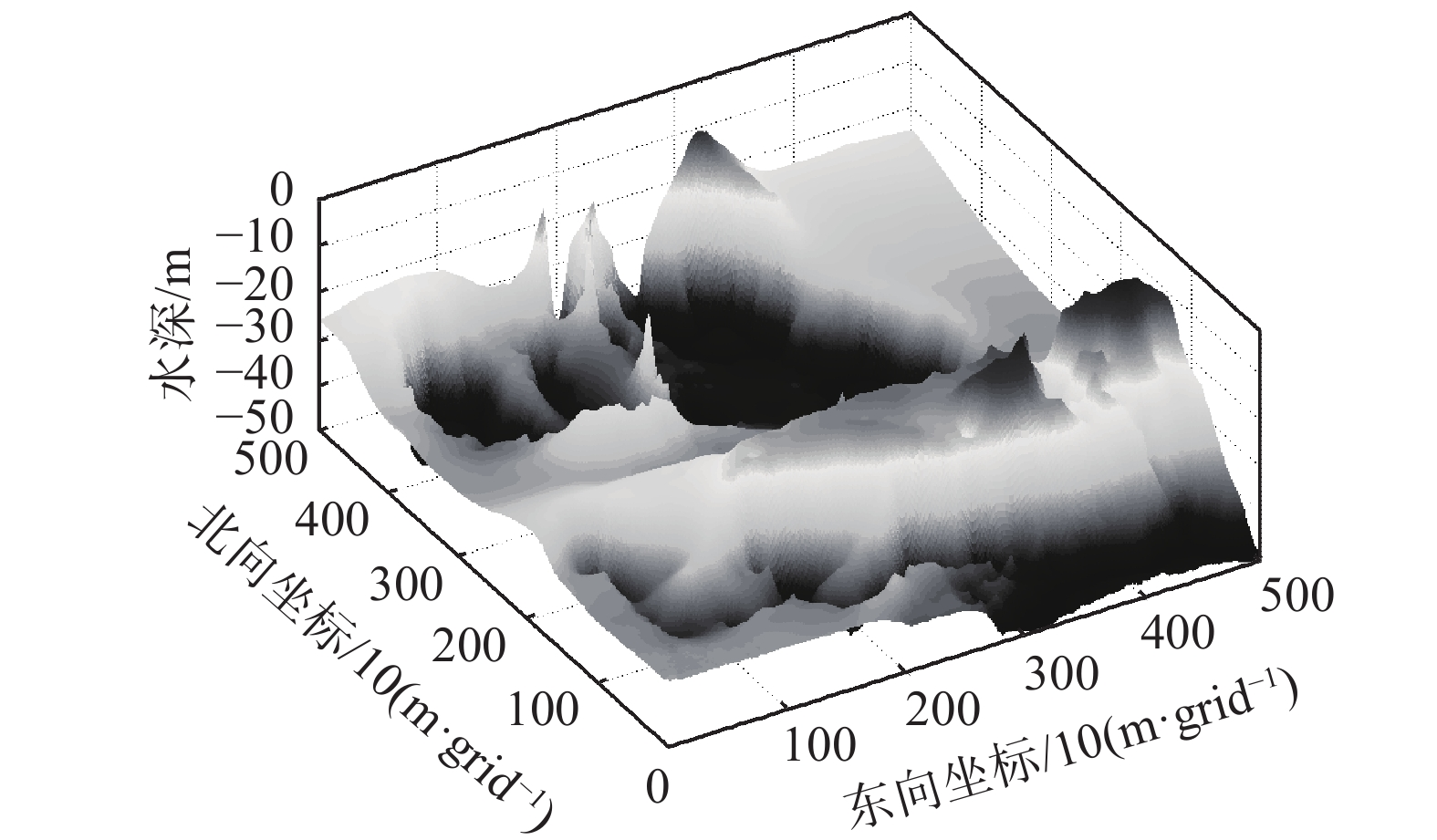Download: 图 5 网格化后的数字地形图 Fig. 5 DTM after gridding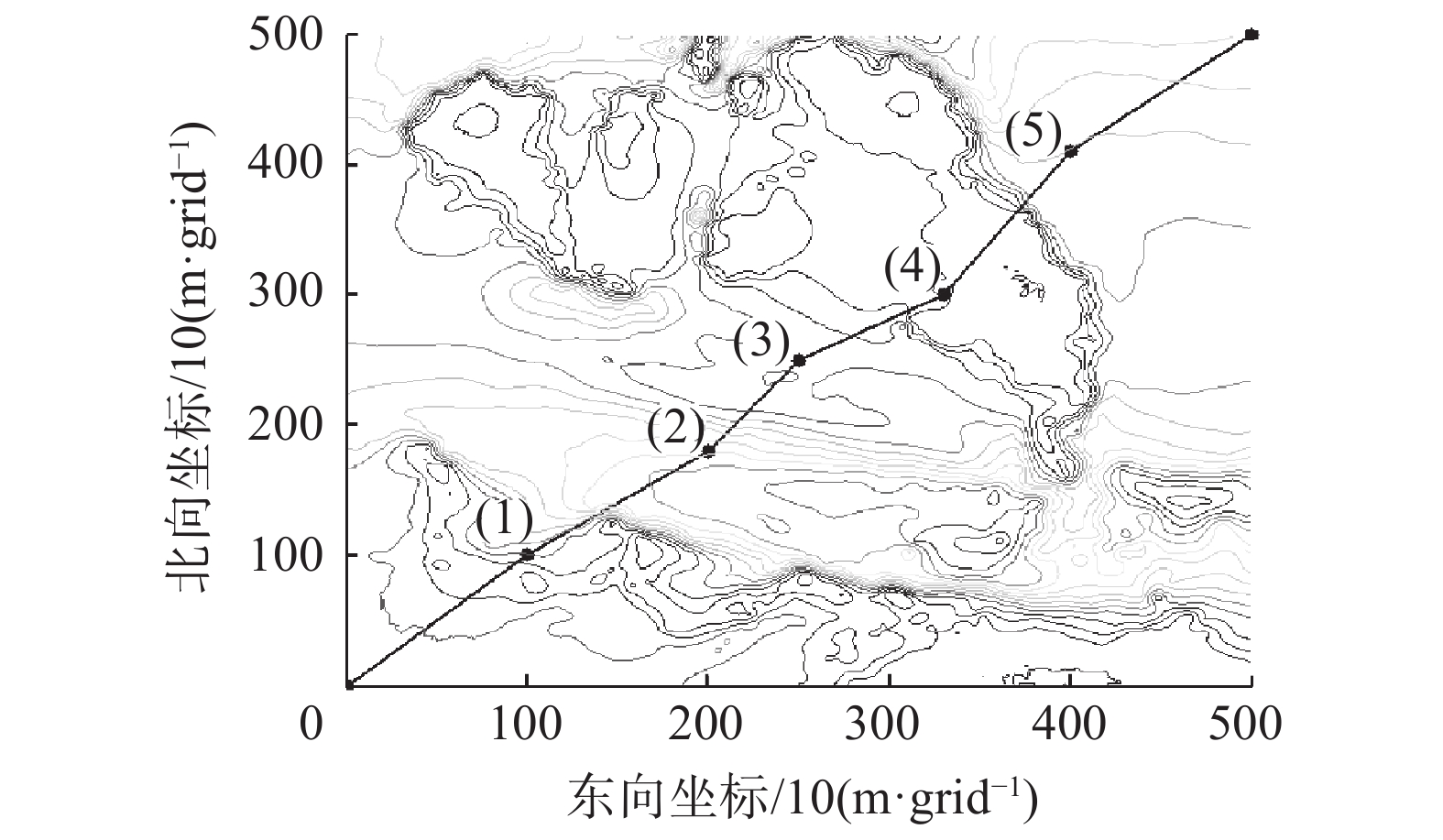Download: 图 6 航行路径和匹配区域 Fig. 6 Navigation path and matching area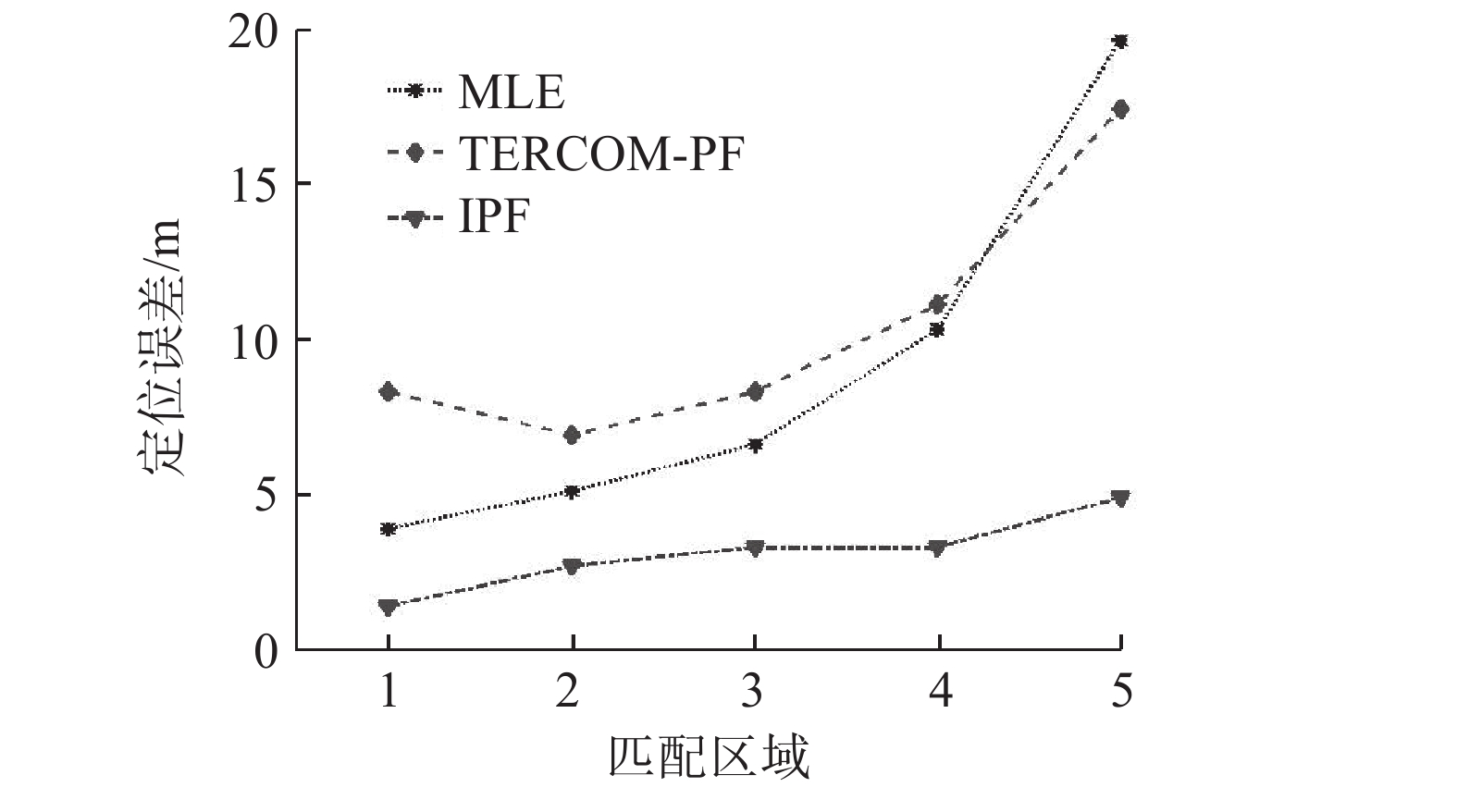Download: 图 7 算法对比仿真结果 Fig. 7 Simulation results of algorithm comparison表 1 匹配区域内的地形熵 Tab.1 Terrain entropy in the matching area
3.3 回放式仿真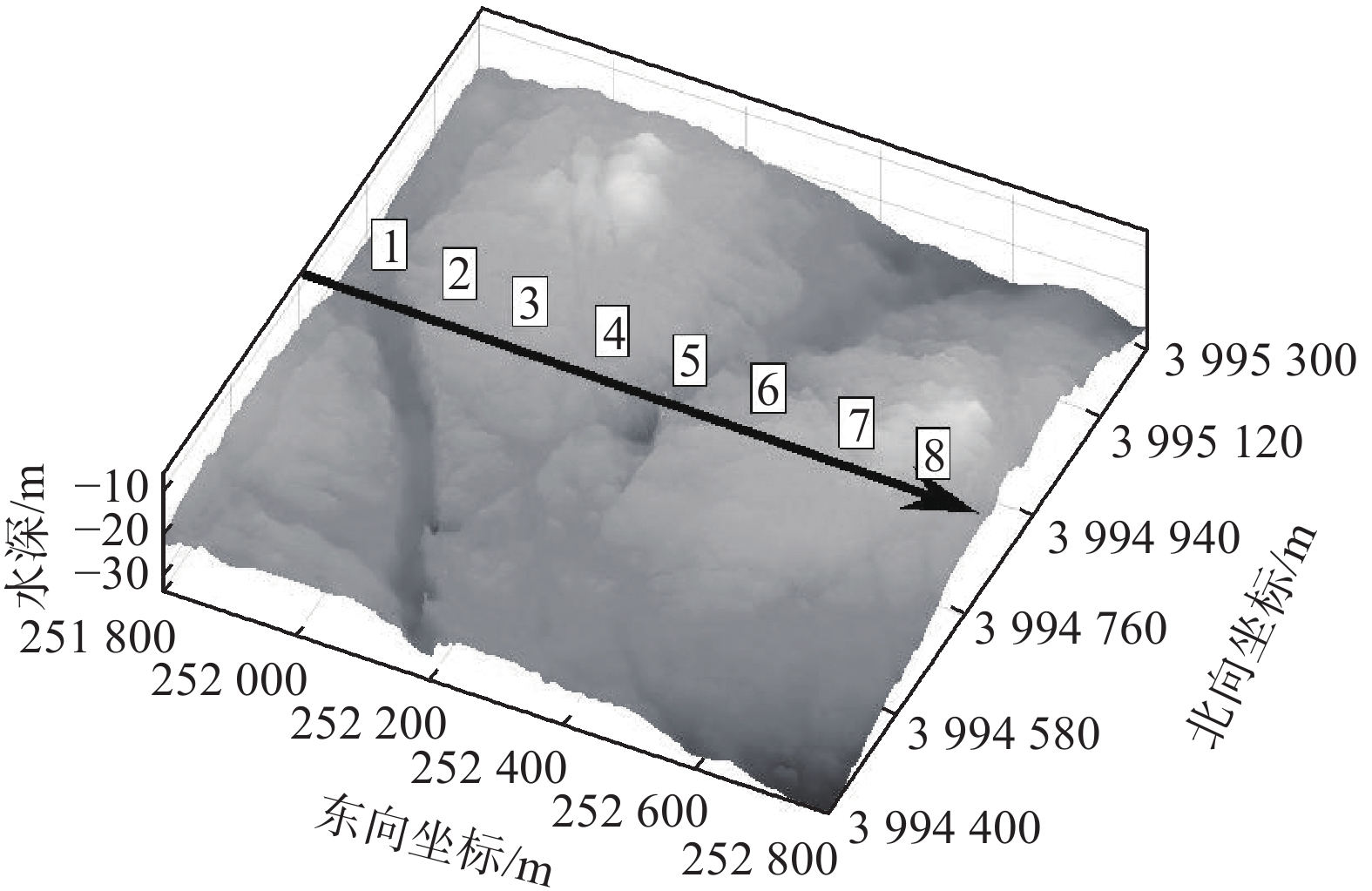Download: 图 8 DTM和独立多波束测线 Fig. 8 DTM and independent multi-beam surveying line表 2 匹配区域内的地形熵 Tab.2 Terrain entropy in the matching area

1) 设定仿真中的粒子数为2 000，选择不同波束组合进行仿真实验，仿真结果如图9所示。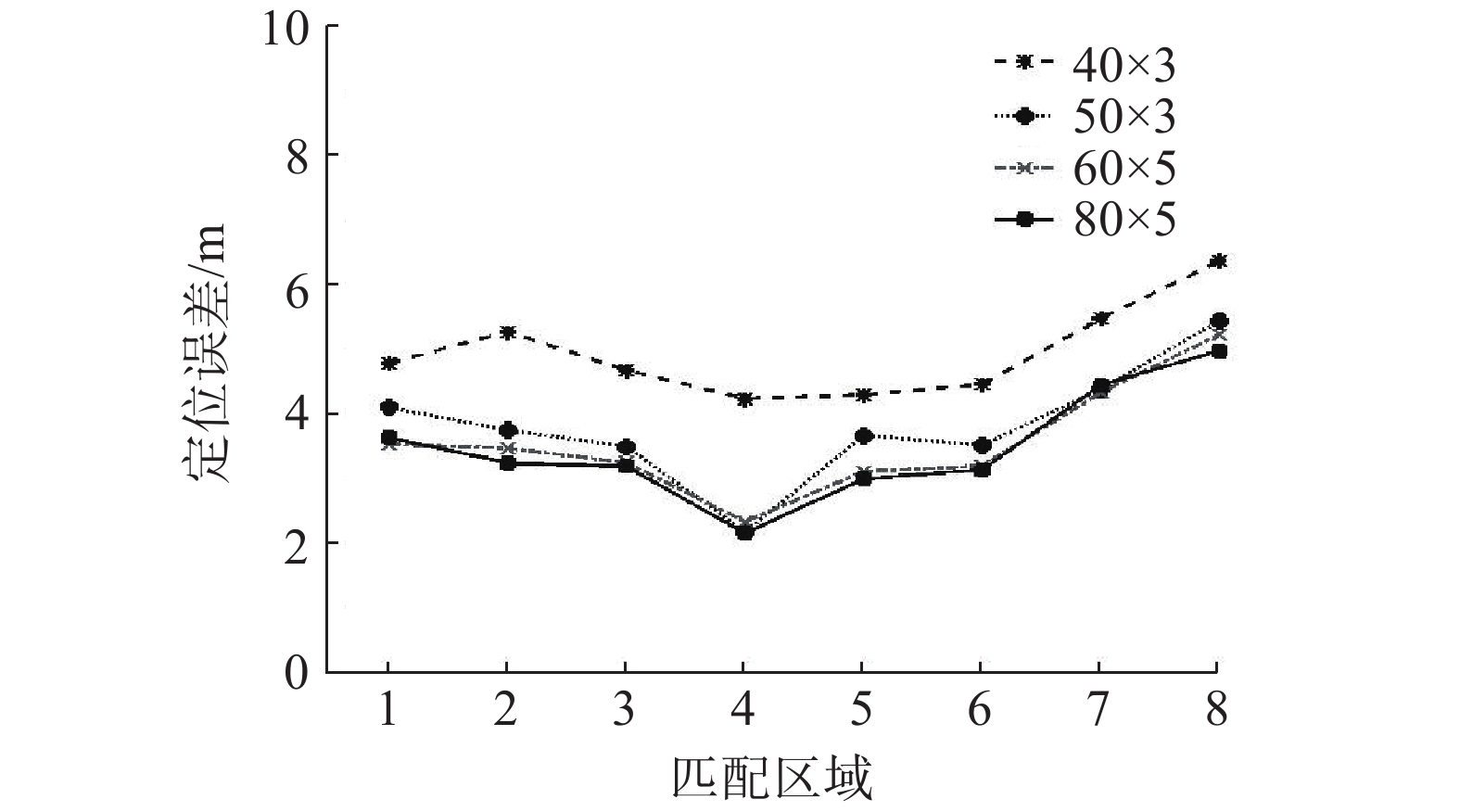Download: 图 9 不同波束组合条件下的仿真结果 Fig. 9 Simulation results based on different beam combination conditions

2) 设定仿真中的波束组合为 $60 \times 5$ ，选择不同粒子数目进行仿真实验，仿真结果如图10所示。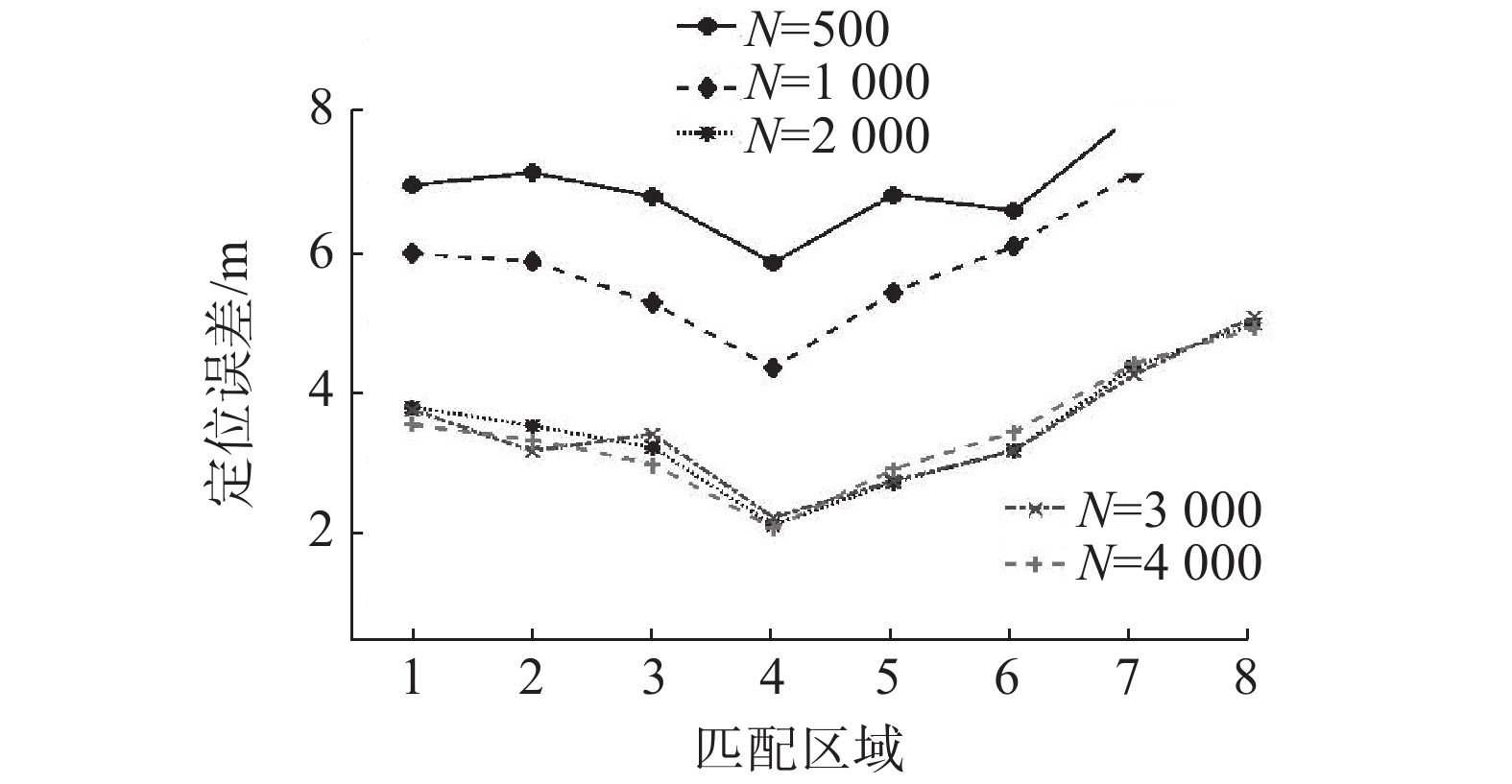Download: 图 10 不同粒子数下的仿真结果 Fig. 10 Simulation results based on different number of particles

4 结束语

  徐玉如, 庞永杰, 甘永, 等. 智能水下机器人技术展望[J]. 智能系统学报, 2006, 1(1): 9-16. XU Yuru, PANG Yongjie, GAN Yong, et al. AUV-State-of-the-art and prospect[J]. CAAI transactions on intelligent systems, 2006, 1(1): 9-16. (0)  潘光, 宋保维, 黄桥高, 等. 水下无人系统发展现状及其关键技术[J]. 水下无人系统学报, 2017, 25(2): 44-51. PAN Guang, SONG Baowei, HUANG Qiaogao, et al. Development and key techniques of unmanned undersea system[J]. Journal of unmanned undersea systems, 2017, 25(2): 44-51. (0)  BRITO M P, LEWIS R S, BOSE N, et al. Adaptive autonomous underwater vehicles: an assessment of their effectiveness for oceanographic applications[J]. IEEE transactions on engineering management, 2019, 66(1): 98-111. DOI:10.1109/TEM.2018.2805159 (0)  PAULL L, SAEEDI S, SETO M, et al. AUV navigation and localization: a review[J]. IEEE journal of oceanic engineering, 2014, 39(1): 131-149. DOI:10.1109/JOE.2013.2278891 (0)  NISHIDA Y, URA T, NAKATANI T, et al. Autonomous underwater vehicle "Tuna-Sand" for image observation of the seafloor at a low altitude[J]. Journal of robotics and mechatronics, 2014, 26(4): 519-521. DOI:10.20965/jrm.2014.p0519 (0)  TEIXEIRA F C, QUINTAS J, MAURYA P, et al. Robust particle filter formulations with application to terrain-aided navigation[J]. International journal of adaptive control and signal processing, 2017, 31(4): 608-651. DOI:10.1002/acs.2692 (0)  MELO J, MATOS A. Survey on advances on terrain based navigation for autonomous underwater vehicles[J]. Ocean engineering, 2017, 139: 250-264. DOI:10.1016/j.oceaneng.2017.04.047 (0)  NYGREN I, JANSSON M. Terrain navigation for underwater vehicles using the correlator method[J]. IEEE journal of oceanic engineering, 2004, 29(3): 906-915. DOI:10.1109/JOE.2004.833222 (0)  陈小龙, 庞永杰, 李晔, 等. 基于极大似然估计的AUV水下地形匹配定位方法[J]. 机器人, 2012, 34(5): 559-565. CHEN Xiaolong, PANG Yongjie, LI Ye, et al. Underwater terrain matching positioning method based on MLE for AUV[J]. Robot, 2012, 34(5): 559-565. DOI:10.3724/SP.J.1218.2012.00559 (0)  LI Ye, WANG Rupeng, CHEN Pengyun, et al. Terrain matching positioning method based on node multi-information fusion[J]. The journal of navigation, 2017, 70(1): 82-100. DOI:10.1017/S0373463316000369 (0)  占荣辉, 张军, 欧建平, 等. 非线性滤波理论与目标跟踪应用[M]. 北京: 国防工业出版社, 2013. ZHAN Ronghui, ZHANG Jun, OU Jianping, et al. Nonlinear filtering theory with target tracking application[M]. Beijing: National Defense Industry Press, 2013. (0)  胡士强, 敬忠良. 粒子滤波算法综述[J]. 控制与决策, 2005, 20(4): 361-365, 371. HU Shiqiang, JING Zhongliang. Overview of particle filter algorithm[J]. Control and decision, 2005, 20(4): 361-365, 371. DOI:10.3321/j.issn:1001-0920.2005.04.001 (0)  LI Ye, CHEN Pengyun, DONG Zaopeng. Sensor simulation of underwater terrain matching based on sea chart[C]//Proceedings of International Conference on Computer Science, Environment, Ecoinformatics, and Education. Wuhan, China, 2011. (0)  HAGEN O K, ANONSEN K B. Using terrain navigation to improve marine vessel navigation systems[J]. Marine technology society journal, 2014, 48(2): 45-58. DOI:10.4031/MTSJ.48.2.6 (0)  王华, 晏磊, 钱旭, 等. 基于地形熵和地形差异熵的综合地形匹配算法[J]. 计算机技术与发展, 2007, 17(9): 25-27. WANG Hua, YAN Lei, QIAN Xu, et al. Integration terrain match algorithm based on terrain entropy and terrain variance entropy[J]. Computer technology and development, 2007, 17(9): 25-27. DOI:10.3969/j.issn.1673-629X.2007.09.008 (0)  ZHAO Long, GAO Nan, HUANG Baoqi, et al. A novel terrain-aided navigation algorithm combined with the TERCOM algorithm and particle filter[J]. IEEE sensors journal, 2015, 15(2): 1124-1131. DOI:10.1109/JSEN.2014.2360916 (0)  HAGEN O K. TerrLab- a generic simulation and post-processing tool for terrain referenced navigation[C]//Proceedings of MTS/IEEE Oceans Conference 2006. Boston, USA, 2006. (0)